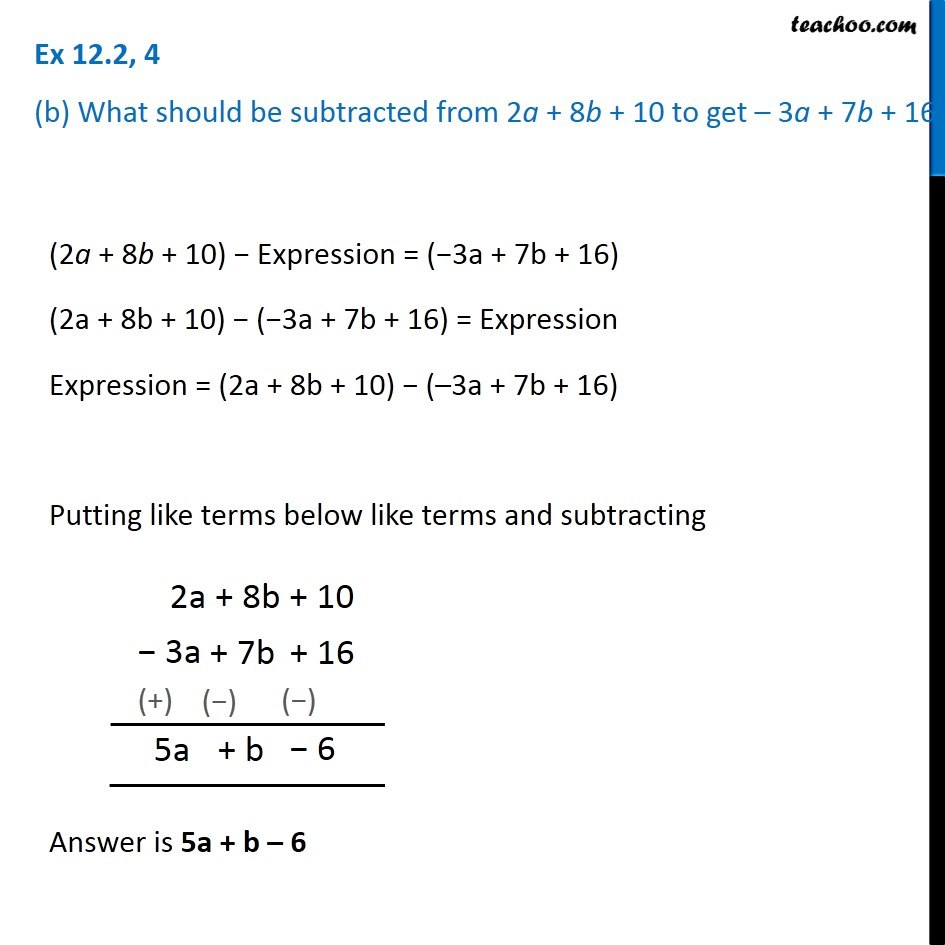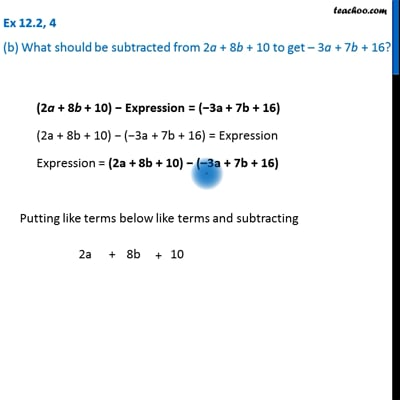Ex 12.2

Chapter 12 Class 7 Algebraic Expressions
Serial order wiseThis video is only available for Teachoo black users

Get live Maths 1-on-1 Classs - Class 6 to 12

### Transcript

Ex 12.2, 4 (b) What should be subtracted from 2a + 8b + 10 to get – 3a + 7b + 16? (2a + 8b + 10) − Expression = (−3a + 7b + 16) (2a + 8b + 10) − (−3a + 7b + 16) = Expression Expression = (2a + 8b + 10) − (–3a + 7b + 16) Putting like terms below like terms and subtracting Answer is 5a + b – 6